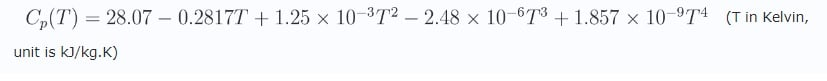## Fluids

•Devank12
Subscriber

I want to define SPECIFIC HEAT of  water that varies with temperature  I have  written a UDF based on the following polynomial equation.  I donot know it is right .If any correction plz inform me. ThanksDEFINE_SPECIFIC_HEAT(specificheat, T, Tref, h, yi)

{

real cp,;

real T = C_T(c,t);

cp = 28.07- (0.2817*T) +(1.25*exp(-3.0) *pow(T,2.))-(2.48*exp(-6.0) *pow(T,3.))+(1.857*exp(-9.0) *pow(T,4.))

C_UDMI(c,t,0) = cp;

return cp;

}

•DrAmine
Ansys Employee

Help your self by using debugging messages. You have started with writing into UDMI's that if fine. You need to use the temperature passed by Fluent. Morever in the manual you have an example for DEFINE_SPECIFIC_HEAT. Actually you don't need an UDF as you can use the polynomial option there.

•RagnorakMan
Subscriber

Hi Amine, would you know what type of units are used for a specific heat capacity based UDF? I'm trying to define a solid with the UDF posted by Devank and I've noticed that his units are kJ/kgK whereas the standard units within Fluent are J/kgK. Your advice will be helpful

•Rob
Ansys Employee

It'll use SI units: likely J/kg K.